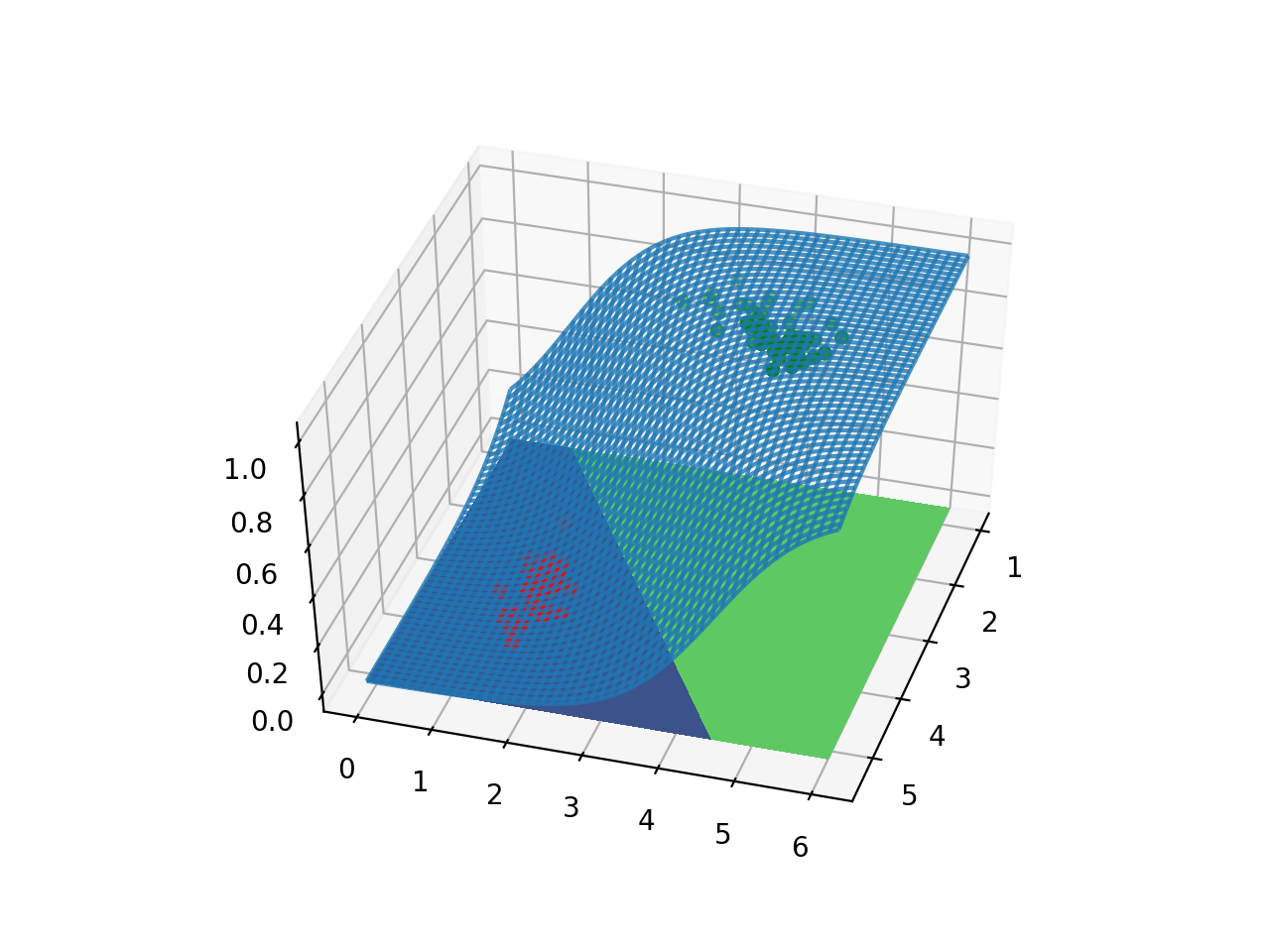## Sigmoid 函数的作用## 逻辑回归是线性模型？## 如何实现非线性？## 损失函数

$$J(\theta) = \frac{1}{m}\sum_{i=1}^{m}{\frac{1}{2} (h_\theta(x^{(i)} ) - y^{(i)} )^2}$$$$J(\theta) = \frac{1}{m}\sum_{i=1}^{m}{ Cost( h_\theta (x^{(i)}) , y)} \\ Cost( h_\theta (x) , y) = \begin{cases} -\log(h_\theta(x)), & \text{if}\ y = 1 \\ -\log(1 - h_\theta(x)), & \text{if}\ y = 0 \end{cases}$$

$$J(\theta) = - \frac{1}{m}\Big[\sum_{i=1}^{m}{ y^{(i)} \log h_{\theta} ( x^{(i)} ) + (1-y^{(i)} ) \log (1-h_\theta(x^{(i)} ) )}\Big]$$## 正则化

$$J(\theta) = - \frac{1}{m}\Big[\sum_{i=1}^{m}{ y^{(i)} \log h_{\theta} ( x^{(i)} ) + (1-y^{(i)} ) \log (1-h_\theta(x^{(i)} ) )}\Big] + \lambda \sum_{j=1}^{m}{( \theta_j^2 )}$$$\lambda$ norm of $\theta$
0 305.21
0.001 75.36
0.01 29.71
0.1 10.02

## 特征缩放## 偏置